# wyz.code.rdoc use cases

#### Last update 2020 - Q1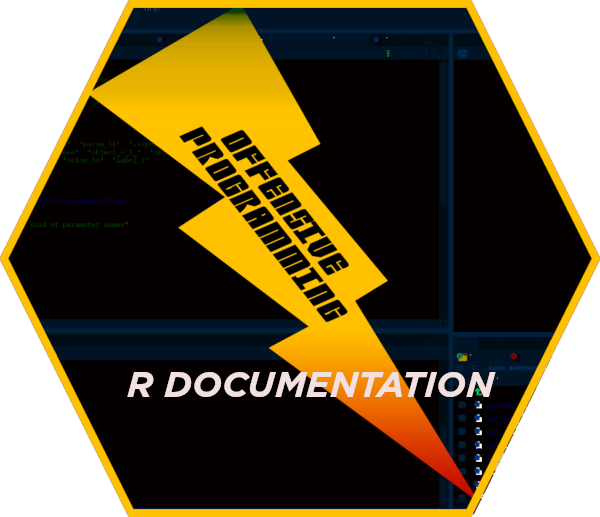This use-case vignette is dedicated to some common manual page use case generation using package wyz.code.rdoc.

It may make sense for newcommers to read the tutorial vignette prior to read this vignette.

# 1 Manual page generation from a function

## 1.1 Standard R function

I will consider function append from base package as example. Any other R function may work as well.

### 1.1.1 code

``````b <- beautify()
examples <- list(
function() { append(1:5, 0:1, after = 3) }
)

ic <- InputContext(NULL, 'append')
pc <- ProcessingContext(
extraneous_l = list(
title = 'Vector Merging',
description = sentensize('add elements to a vector'),
details = paste('If the parameter', b\$code('after'), 'is higher than' ,
b\$code('x'), 'length,\n then insertion is done at the',
'end of the data structure'),
references = paste('Becker, R. A., Chambers, J. M. and Wilks, A. R. (1988)',
'The New S Language. Wadsworth & Brooks/Cole.'),
examples = convertExamples(examples, captureOutput_b_1n = FALSE)
),
postProcessing_l = list(
arguments = function(content_s) {
s <- sub('XXX_001', sentensize('the vector the values are to be appended to'),
content_s, fixed = TRUE)
s <- sub('XXX_002', sentensize('to be included in the modified vector'), s, fixed = TRUE)
s <- sub('XXX_003',
'a subscript, after which the values are to be appended',
s, fixed = TRUE)
s
}
)
)
td <- tempdir()
gc <- GenerationContext(td, overwrite = TRUE)
rv <- produceManualPage(ic, pc, gc)
File /tmp/Rtmphizv7q/append.Rd passes standard documentation checks ``````

NOTA BENE: Embedded new line characters in previous text are present for vignette format purpose only here, in particular to avoid horizontal scrollbars and to ease reading. Indeed, sometimes you will have to do so, to avoid producing too long lines in R documentations files, as this will generate a warning when running R CMD check. If you plan to publish your package on CRAN, you will have to resolve such warning cases.

### 1.1.2 generated .Rd file

``````readLines(rv\$context\$filename)
 "\\name{append}"
 "\\alias{append}"
 "\\title{Vector Merging}"
 "\\description{"
 "Add elements to a vector."
 "}"
 "\\usage{"
 "append(x, values, after = length(x))"
 "}"
 "\\arguments{"
 "\\item{x}{The vector the values are to be appended to.}"
 "\\item{values}{To be included in the modified vector.}"
 "\\item{after}{a subscript, after which the values are to be appended}"
 "}"
 "\\details{"
 "If the parameter \\code{after} is higher than \\code{x} length,"
 " then insertion is done at the end of the data structure"
 "}"
 "\\references{"
 "Becker, R. A., Chambers, J. M. and Wilks, A. R. (1988) The New S Language. Wadsworth & Brooks/Cole."
 "}"
 "\\examples{"
 "# ------- example 1 -------"
 "append(1:5, 0:1, after = 3) "
 ""
 "}"
 "\\keyword{function}"
 "\\encoding{UTF-8}"                                                                                  ``````

### 1.1.3 generated content as picture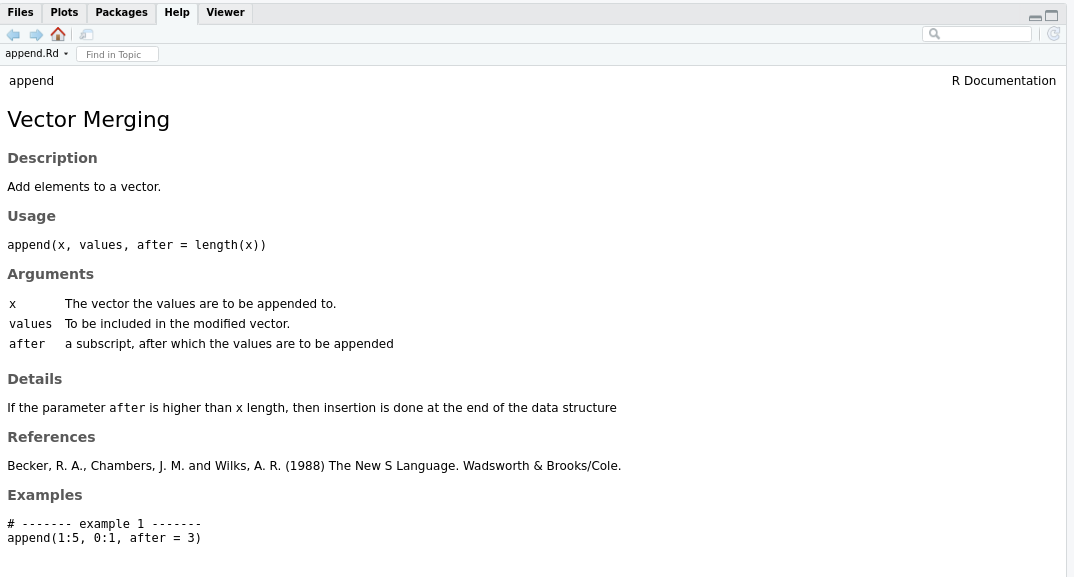generated append documentation

### 1.1.4 original content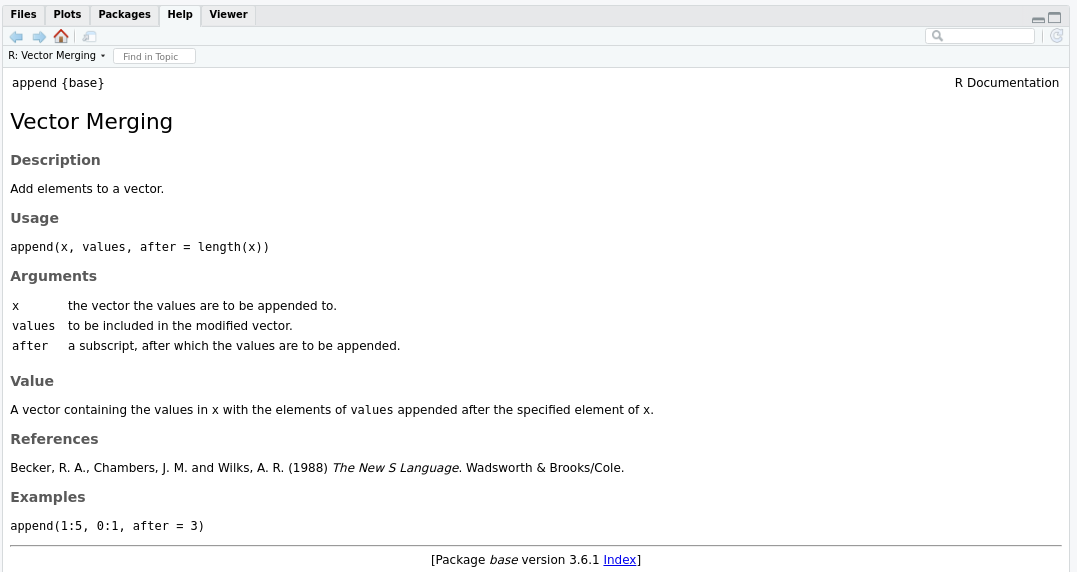base R append documentation

## 1.2 Semantic naming function

Let’s consider following function, respectful of semantic naming as defined by offensive programming. Refer to Offensive programming book to get introduction and advanced knowledge on offensive programming advantages, practice and ecosystem.

``````iterateOverSet <- function(set_s, enforceUniqueness_b_1 = TRUE) {
NULL
}``````

The body is set to NULL for demonstration purpose and to prove that it does not interfere with documentation generation.

### 1.2.1 code

``````examples <- list(
function() { iterateOverSet(sample(LETTERS, 35, TRUE), FALSE) },
function() { iterateOverSet(as.character(1:9)) }
)
ic <- InputContext(NULL, 'iterateOverSet')
pc <- ProcessingContext(
extraneous_l = list(
examples = convertExamples(examples, captureOutput_b_1n = FALSE)
)
)
rv_op <- produceManualPage(ic, pc, gc)
File /tmp/Rtmphizv7q/iterateOverSet.Rd passes standard documentation checks ``````

### 1.2.2 generated .Rd file

``````readLines(rv_op\$context\$filename)
 "\\name{iterateOverSet}"
 "\\alias{iterateOverSet}"
 "\\title{Function iterateOverSet}"
 "\\description{"
 "Use this function to iterate over set."
 "}"
 "\\usage{"
 "iterateOverSet(set_s, enforceUniqueness_b_1 = TRUE)"
 "}"
 "\\arguments{"
 "\\item{set_s}{an unconstrained \\emph{\\code{vector}} of \\emph{\\code{string}} values representing the set}"
 "\\item{enforceUniqueness_b_1}{a single \\emph{\\code{boolean}} value representing the enforce uniqueness}"
 "}"
 "\\examples{"
 "# ------- example 1 -------"
 "iterateOverSet(sample(LETTERS, 35, TRUE), FALSE) "
 ""
 "# ------- example 2 -------"
 "iterateOverSet(as.character(1:9)) "
 ""
 "}"
 "\\keyword{function}"
 "\\encoding{UTF-8}"                                                                                           ``````

### 1.2.3 generated content as picture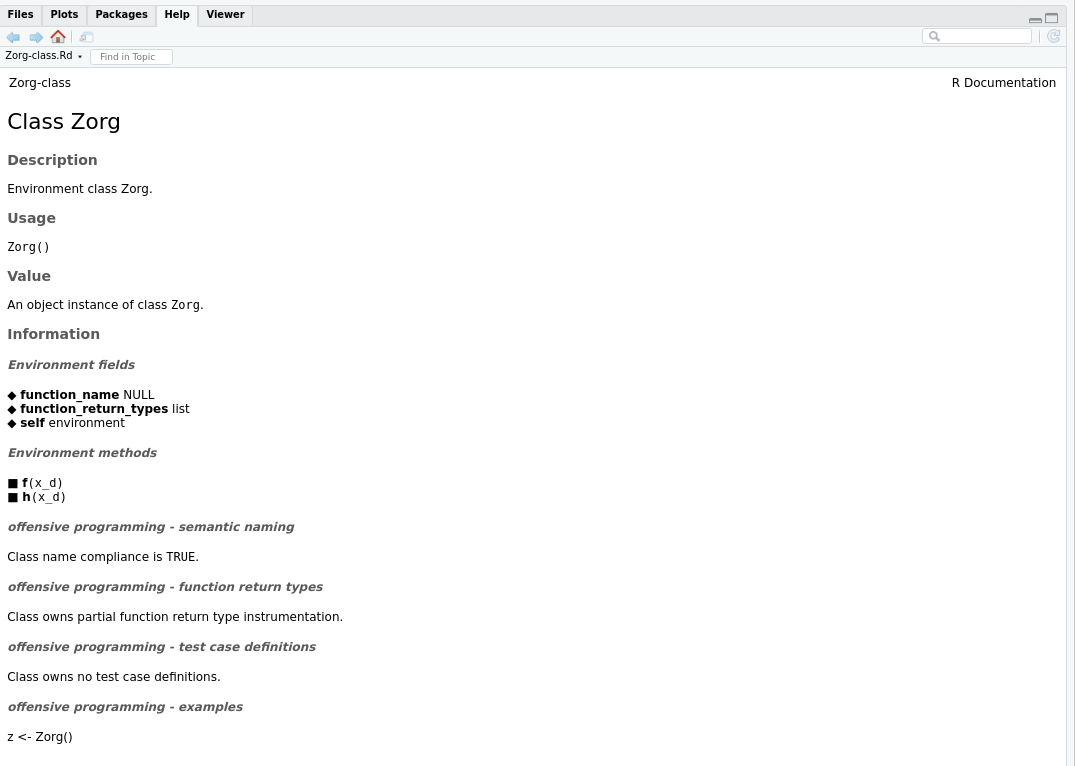generated append documentation

### 1.2.4 Main differences

Main differences with standard R function generation are, using semantic naming scheme, you do not have to

1. provide content for arguments. It is automatically deduced from the semantic name you use
2. provide a title. It is deduced from the function name.
3. provide a description. It is also deduced from the function name.

You can still change or adapt provided content by a post processing whenever and wherever needed to meet sharper documentation level.

# 2 Manual page generation from an environment object

## 2.1 Standard R object

I will consider class named MeltingPot_Env to produce an object based on an environment containing several functions.

### 2.1.1 code

``````source(findFilesInPackage('classes', 'wyz.code.offensiveProgramming'))
ic <- InputContext(MeltingPot_Env())
rv <- produceManualPage(ic, generationContext = gc)
File /tmp/Rtmphizv7q/MeltingPot_Env-class.Rd passes standard documentation checks ``````

NOTA BENE: I use default processing context here. This means, only defaultly valuable documentation sections will be filled-in.

### 2.1.2 generated .Rd file

``````readLines(rv\$context\$filename)
 "\\name{MeltingPot_Env-class}"
 "\\docType{class}"
 "\\alias{MeltingPot_Env-class}"
 "\\alias{MeltingPot_Env}"
 "\\title{Class MeltingPot_Env}"
 "\\description{"
 "Environment class MeltingPot_Env."
 "}"
 "\\usage{"
 "MeltingPot_Env()"
 "}"
 "\\value{"
 "An \\emph{\\code{object}} instance of \\emph{\\code{environment}} class \\emph{\\bold{\\code{\\link{MeltingPot_Env}}}}."
 "}"
 "\\section{Information}{"
 "\\subsection{Environment fields}{"
 "\\tabular{lll}{"
 "\\ifelse{latex}{\\out{\$\\mapsto\$}}{\\ifelse{html}{\\out{&#x25C6;}}{\\\\u25c6}} \\tab \\bold{self} \\tab environment \\cr"
 "}"
 "}"
 "\\subsection{Environment methods}{"
 "\\cr \\ifelse{latex}{\\out{\$\\rightarrow\$}}{\\ifelse{html}{\\out{&#x25A0;}}{\\\\u25a0}} \\bold{add3length}\\code{(x_i_3l, y_i_3l = 1:3)}\\cr"
 "\\ifelse{latex}{\\out{\$\\rightarrow\$}}{\\ifelse{html}{\\out{&#x25A0;}}{\\\\u25a0}} \\bold{blabla}\\code{(a_s = \"bla\", b_s = c(\"bli\", \"blo\", \"blu\"))}\\cr"
 "\\ifelse{latex}{\\out{\$\\rightarrow\$}}{\\ifelse{html}{\\out{&#x25A0;}}{\\\\u25a0}} \\bold{divideByZero}\\code{(x_n)}\\cr"
 "\\ifelse{latex}{\\out{\$\\rightarrow\$}}{\\ifelse{html}{\\out{&#x25A0;}}{\\\\u25a0}} \\bold{echo}\\code{(x_s = \"hello world\")}\\cr"
 "\\ifelse{latex}{\\out{\$\\rightarrow\$}}{\\ifelse{html}{\\out{&#x25A0;}}{\\\\u25a0}} \\bold{generateError}\\code{()}\\cr"
 "\\ifelse{latex}{\\out{\$\\rightarrow\$}}{\\ifelse{html}{\\out{&#x25A0;}}{\\\\u25a0}} \\bold{generateWarning}\\code{()}"
 "}"
 "\\subsection{offensive programming - semantic naming}{"
 "Class name compliance is \\emph{\\code{FALSE}}."
 "}"
 "\\subsection{offensive programming - function return types}{"
 "Class owns no function return type instrumentation."
 "}"
 "\\subsection{offensive programming - test case definitions}{"
 "Class owns no test case definitions."
 "}"
 "\\subsection{offensive programming - examples}{"
 "mpe <- MeltingPot_Env()"
 "}"
 "}"
 "\\keyword{class}"
 "\\encoding{UTF-8}"                                                                                                                                               ``````

### 2.1.3 generated content as picture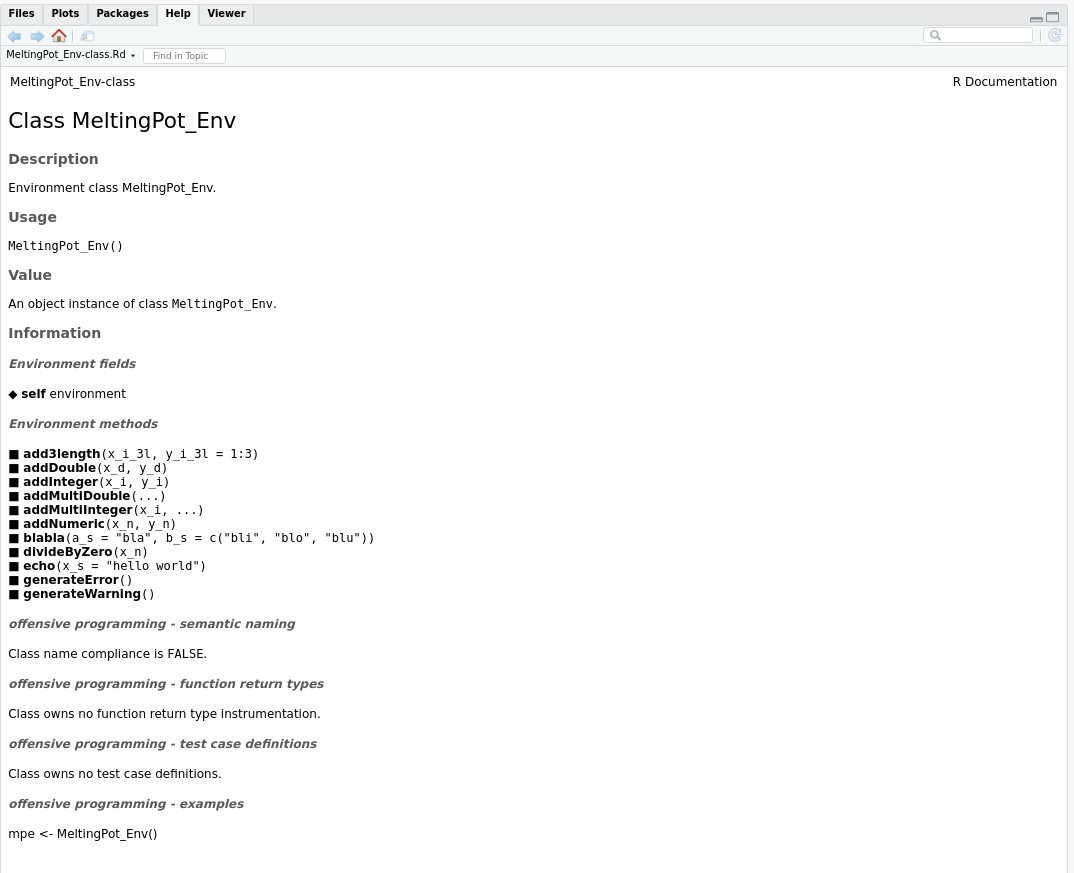generated append documentation

## 2.2 Offensive programming object

I will consider class named Zorg to produce an object based on an environment containing several functions. This class is partially offensive programming instrumented.

### 2.2.1 code

``````ic <- InputContext(Zorg())
rv <- produceManualPage(ic, generationContext = gc)
File /tmp/Rtmphizv7q/Zorg-class.Rd passes standard documentation checks ``````

### 2.2.2 generated .Rd file

``````readLines(rv\$context\$filename)
 "\\name{Zorg-class}"
 "\\docType{class}"
 "\\alias{Zorg-class}"
 "\\alias{Zorg}"
 "\\title{Class Zorg}"
 "\\description{"
 "Environment class Zorg."
 "}"
 "\\usage{"
 "Zorg()"
 "}"
 "\\value{"
 "An \\emph{\\code{object}} instance of \\emph{\\code{environment}} class \\emph{\\bold{\\code{\\link{Zorg}}}}."
 "}"
 "\\section{Information}{"
 "\\subsection{Environment fields}{"
 "\\tabular{lll}{"
 "\\ifelse{latex}{\\out{\$\\mapsto\$}}{\\ifelse{html}{\\out{&#x25C6;}}{\\\\u25c6}} \\tab \\bold{function_name} \\tab NULL \\cr"
 "\\ifelse{latex}{\\out{\$\\mapsto\$}}{\\ifelse{html}{\\out{&#x25C6;}}{\\\\u25c6}} \\tab \\bold{function_return_types} \\tab list \\cr"
 "\\ifelse{latex}{\\out{\$\\mapsto\$}}{\\ifelse{html}{\\out{&#x25C6;}}{\\\\u25c6}} \\tab \\bold{self} \\tab environment \\cr"
 "}"
 "}"
 "\\subsection{Environment methods}{"
 "\\cr \\ifelse{latex}{\\out{\$\\rightarrow\$}}{\\ifelse{html}{\\out{&#x25A0;}}{\\\\u25a0}} \\bold{f}\\code{(x_d)}\\cr"
 "\\ifelse{latex}{\\out{\$\\rightarrow\$}}{\\ifelse{html}{\\out{&#x25A0;}}{\\\\u25a0}} \\bold{h}\\code{(x_d)}"
 "}"
 "\\subsection{offensive programming - semantic naming}{"
 "Class name compliance is \\emph{\\code{TRUE}}."
 "}"
 "\\subsection{offensive programming - function return types}{"
 "Class owns partial function return type instrumentation."
 "\\tabular{rr}{\\code{function_name} \\tab \\code{return_value}\\cr"
 ""
 "\\bold{f} \\tab x_d \\cr"
 "}"
 "}"
 "\\subsection{offensive programming - test case definitions}{"
 "Class owns no test case definitions."
 "}"
 "\\subsection{offensive programming - examples}{"
 "z <- Zorg()"
 "}"
 "}"
 "\\keyword{class}"
 "\\encoding{UTF-8}"                                                                                                                 ``````

### 2.2.3 generated content as picture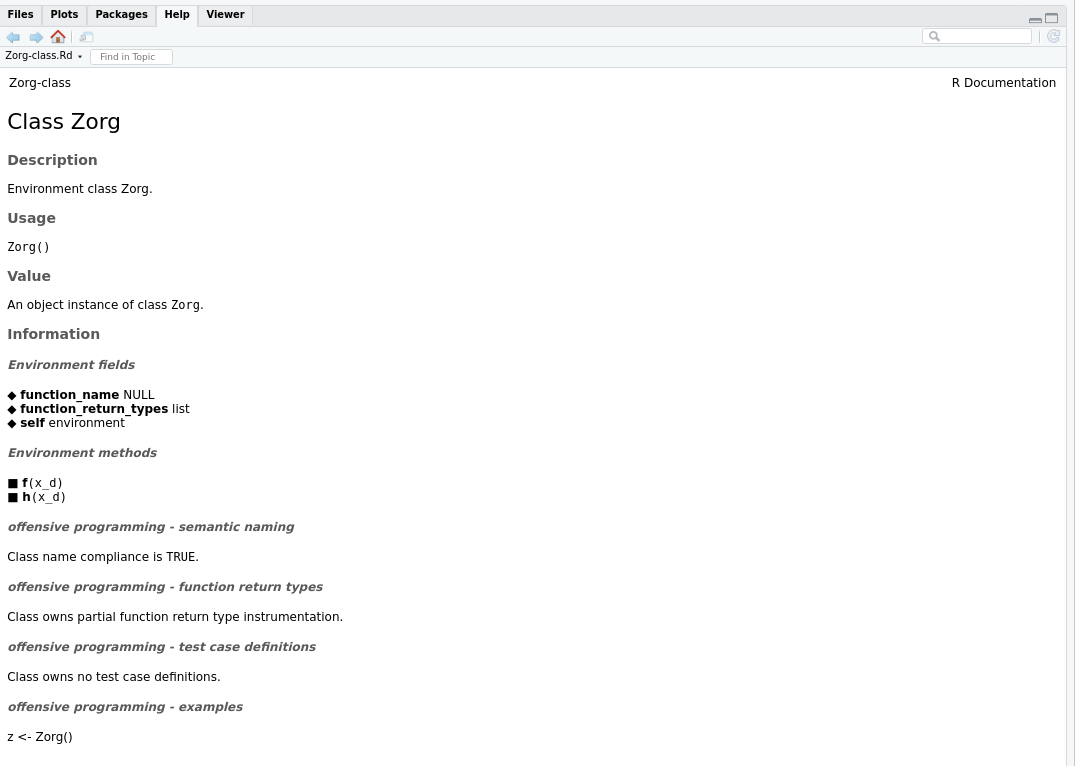generated append documentation

### 2.2.4 Main differences

There exists only very few differences between standard R and offensive programming class documentation generation. Using the later allows to benefit from instrumentation and allows to run dynamic checks instead of static checks.

# 3 Manual page generation from an S3 object

## 3.1 Standard R object

I will consider class named Bu_S3 to produce an object based on S3 class scheme containing several functions.

### 3.1.1 code

``````ic <- InputContext(Bu_S3())
rv <- produceManualPage(ic, generationContext = gc)
File /tmp/Rtmphizv7q/Bu_S3-class.Rd passes standard documentation checks ``````

### 3.1.2 generated .Rd file

``````readLines(rv\$context\$filename)
 "\\name{Bu_S3-class}"
 "\\docType{class}"
 "\\alias{Bu_S3-class}"
 "\\alias{Bu_S3}"
 "\\title{Class Bu_S3}"
 "\\description{"
 "S3 class Bu_S3."
 "}"
 "\\usage{"
 "Bu_S3(x_l = list(l = letters, d = 0:9))"
 "}"
 "\\arguments{"
 "\\item{x_l}{an unconstrained \\emph{\\code{list}} representing the x}"
 "}"
 "\\value{"
 "An \\emph{\\code{object}} instance of \\emph{\\code{S3}} class \\emph{\\bold{\\code{\\link{Bu_S3}}}}."
 "}"
 "\\section{Information}{"
 "\\subsection{S3 fields}{"
 "\\tabular{lll}{"
 "\\ifelse{latex}{\\out{\$\\mapsto\$}}{\\ifelse{html}{\\out{&#x25C6;}}{\\\\u25c6}} \\tab \\bold{d} \\tab integer \\cr"
 "\\ifelse{latex}{\\out{\$\\mapsto\$}}{\\ifelse{html}{\\out{&#x25C6;}}{\\\\u25c6}} \\tab \\bold{l} \\tab character \\cr"
 "}"
 "}"
 "\\subsection{S3 methods}{"
 "\\cr \\ifelse{latex}{\\out{\$\\rightarrow\$}}{\\ifelse{html}{\\out{&#x25A0;}}{\\\\u25a0}} \\bold{alpha}\\code{(x, msg_s = \"\", ...)}\\cr"
 "\\ifelse{latex}{\\out{\$\\rightarrow\$}}{\\ifelse{html}{\\out{&#x25A0;}}{\\\\u25a0}} \\bold{print}\\code{(x, ...)}"
 "}"
 "\\subsection{offensive programming - semantic naming}{"
 "Class name compliance is \\emph{\\code{FALSE}}."
 "}"
 "\\subsection{offensive programming - function return types}{"
 "Class owns no function return type instrumentation."
 "}"
 "\\subsection{offensive programming - test case definitions}{"
 "Class owns no test case definitions."
 "}"
 "\\subsection{offensive programming - examples}{"
 "bs <- Bu_S3(x_l = list(l = letters, d = 0:9))"
 "}"
 "}"
 "\\keyword{class}"
 "\\encoding{UTF-8}"                                                                                                                      ``````

### 3.1.3 generated content as picture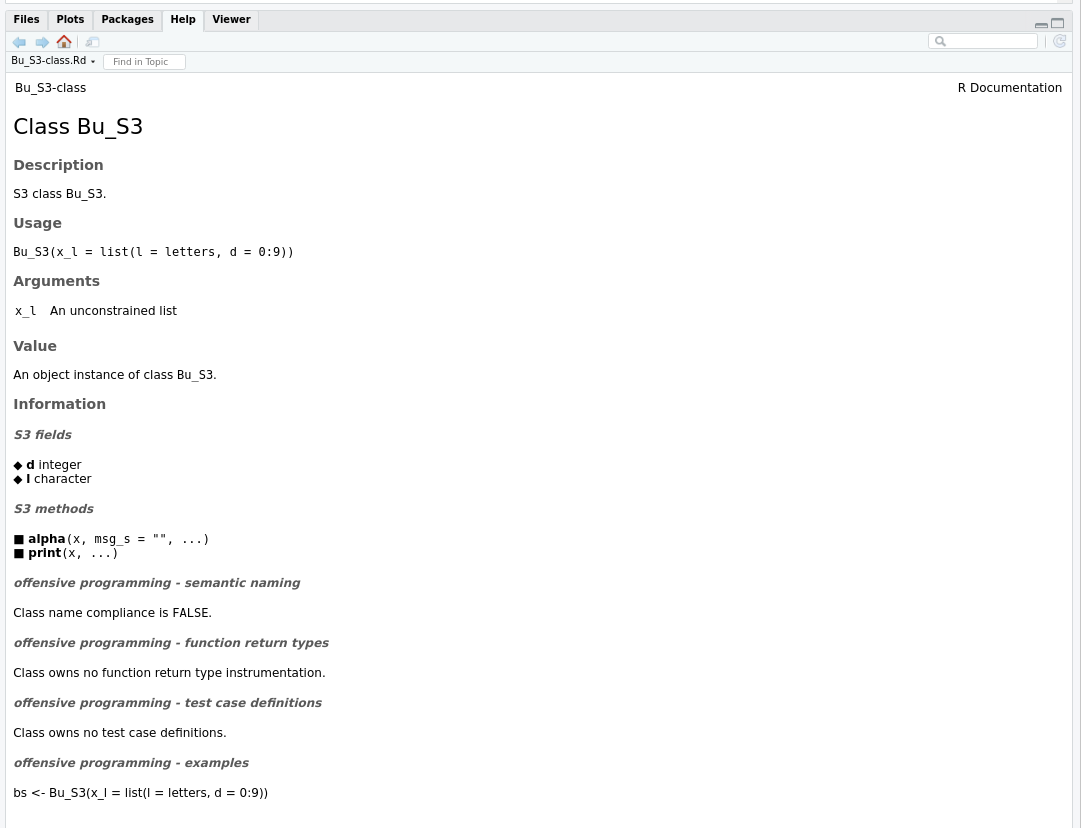generated append documentation

## 3.2 Offensive programming object

I will consider class named Addition_TCFI_Partial_S3 to produce an object based on a S3 class containing several functions. This class is partially offensive programming instrumented.

### 3.2.1 code

``````source(findFilesInPackage('Addition_TCFI_Partial_S3.R', 'wyz.code.offensiveProgramming'))
rv <- produceManualPage(ic, generationContext = gc)
File /tmp/Rtmphizv7q/Addition_TCFI_Partial_S3-class.Rd passes standard documentation checks ``````

### 3.2.2 generated .Rd file

``````readLines(rv\$context\$filename)
 "\\docType{class}"
 "\\description{"
 "}"
 "\\usage{"
 "}"
 "\\value{"
 "}"
 "\\section{Information}{"
 "\\subsection{S3 fields}{"
 "\\tabular{lll}{"
 "\\ifelse{latex}{\\out{\$\\mapsto\$}}{\\ifelse{html}{\\out{&#x25C6;}}{\\\\u25c6}} \\tab \\bold{function_return_types} \\tab list \\cr"
 "\\ifelse{latex}{\\out{\$\\mapsto\$}}{\\ifelse{html}{\\out{&#x25C6;}}{\\\\u25c6}} \\tab \\bold{label} \\tab character \\cr"
 "\\ifelse{latex}{\\out{\$\\mapsto\$}}{\\ifelse{html}{\\out{&#x25C6;}}{\\\\u25c6}} \\tab \\bold{test_case_definitions} \\tab list \\cr"
 "}"
 "}"
 "\\subsection{S3 methods}{"
 "\\cr \\ifelse{latex}{\\out{\$\\rightarrow\$}}{\\ifelse{html}{\\out{&#x25A0;}}{\\\\u25a0}} \\bold{addInteger}\\code{(object_o_1, x_i, y_i)}\\cr"
 "\\ifelse{latex}{\\out{\$\\rightarrow\$}}{\\ifelse{html}{\\out{&#x25A0;}}{\\\\u25a0}} \\bold{generateError}\\code{(object_o_1)}\\cr"
 "\\ifelse{latex}{\\out{\$\\rightarrow\$}}{\\ifelse{html}{\\out{&#x25A0;}}{\\\\u25a0}} \\bold{generateWarning2}\\code{(object_o_1)}"
 "}"
 "\\subsection{offensive programming - semantic naming}{"
 "Class name compliance is \\emph{\\code{FALSE}}."
 "}"
 "\\subsection{offensive programming - function return types}{"
 "Class owns partial function return type instrumentation."
 "\\tabular{rr}{\\code{function_name} \\tab \\code{return_value}\\cr"
 ""
 "\\bold{generateError} \\tab x_er \\cr"
 "}"
 "}"
 "\\subsection{offensive programming - test case definitions}{"
 "Class owns partial test case definitions."
 "\\tabular{rr}{\\code{function_name} \\tab \\code{recorded_tests}\\cr"
 ""
 "\\bold{generateError} \\tab 1 \\cr"
 "}"
 "}"
 "\\subsection{offensive programming - examples}{"
 "\\preformatted{library(\"data.table\")"
 "library(\"wyz.code.offensiveProgramming\")"
 ""
 "tcnum <- 7 # [1,10]"
 ""
 "# standard_R_evaluation mode"
 "se <- EvaluationMode(defineEvaluationMode())"
 "ser <- runTestCase(atcfips, tcnum , se)"
 ""
 "# type_checking_enforcement mode"
 "tc <- EvaluationMode(defineEvaluationMode())"
 "tcr <- runTestCase(atcfips, tcnum, tc)}"
 "}"
 "}"
 "\\keyword{class}"
 "\\encoding{UTF-8}"                                                                                                                           ``````

### 3.2.3 generated content as picture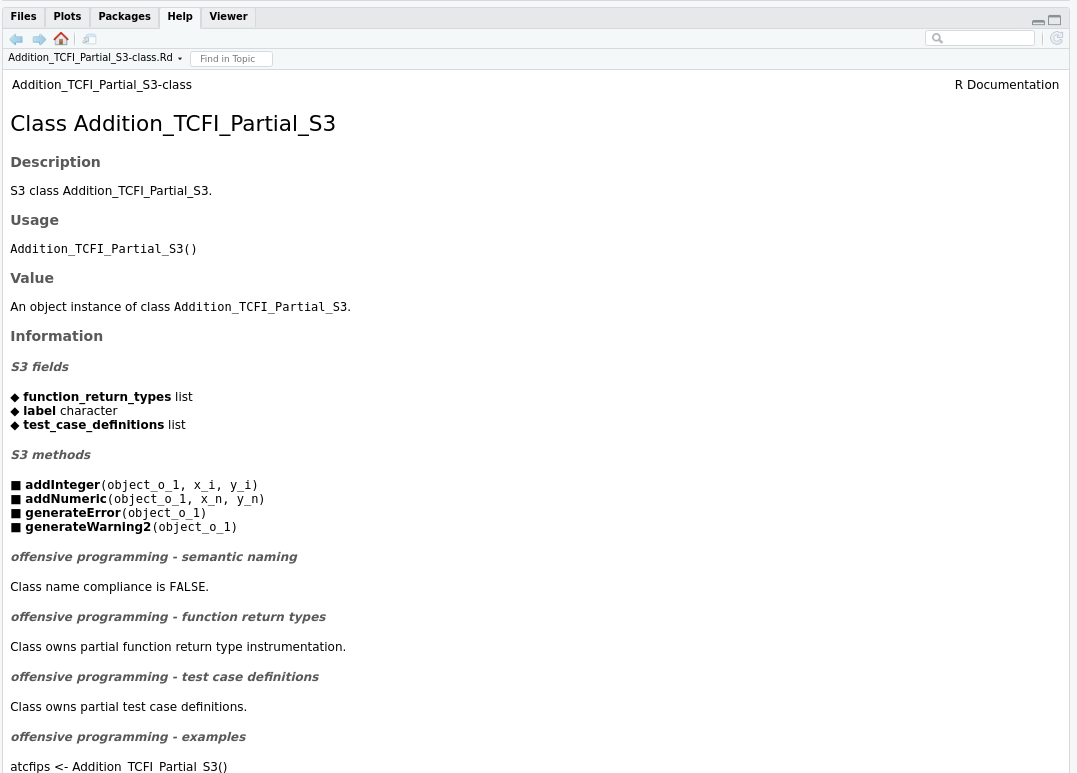generated append documentation

### 3.2.4 Main differences

There exists only very few differences between standard R and offensive programming class documentation generation. Using the later allows to benefit from instrumentation and allows to run dynamic checks instead of static checks.

# 4 Manual page generation from an S4 object

## 4.1 Standard R object

I will consider class named Person_S4 to produce an object based on S4 class scheme containing several functions.

### 4.1.1 code

``````ic <- InputContext(new('Person_S4', name = 'neonira'))
rv <- produceManualPage(ic, generationContext = gc)
File /tmp/Rtmphizv7q/Person_S4-class.Rd passes standard documentation checks ``````

### 4.1.2 generated .Rd file

``````readLines(rv\$context\$filename)
 "\\name{Person_S4-class}"
 "\\docType{class}"
 "\\alias{Person_S4-class}"
 "\\alias{Person_S4}"
 "\\title{Class Person_S4}"
 "\\description{"
 "S4 class Person_S4."
 "}"
 "\\usage{"
 "Person_S4(...)"
 "}"
 "\\arguments{"
 "}"
 "\\value{"
 "An \\emph{\\code{object}} instance of \\emph{\\code{S4}} class \\emph{\\bold{\\code{\\link{Person_S4}}}}."
 "}"
 "\\section{Information}{"
 "\\subsection{S4 fields}{"
 "\\ifelse{latex}{\\out{\$\\mapsto\$}}{\\ifelse{html}{\\out{&#x25C6;}}{\\\\u25c6}} \\bold{name} character\\cr"
 "\\ifelse{latex}{\\out{\$\\mapsto\$}}{\\ifelse{html}{\\out{&#x25C6;}}{\\\\u25c6}} \\bold{age} double"
 "}"
 "\\subsection{S4 methods}{"
 "\\cr \\ifelse{latex}{\\out{\$\\rightarrow\$}}{\\ifelse{html}{\\out{&#x25A0;}}{\\\\u25a0}} \\bold{name}\\code{(o_)}"
 "}"
 "\\subsection{offensive programming - semantic naming}{"
 "Class name compliance is \\emph{\\code{FALSE}}."
 "}"
 "\\subsection{offensive programming - function return types}{"
 "Class owns no function return type instrumentation."
 "}"
 "\\subsection{offensive programming - test case definitions}{"
 "Class owns no test case definitions."
 "}"
 "\\subsection{offensive programming - examples}{"
 "ps <- Person_S4(...)"
 "}"
 "}"
 "\\keyword{class}"
 "\\encoding{UTF-8}"                                                                                               ``````